Samchon Framework for CPP  1.0.0
samchon::library::Math Class Reference

Utility of Math. More...

#include <Math.hpp>

## Static Public Member Functions

static auto E () -> double
Exponent. More...

static auto PI () -> double
��, Number Pi More...

static auto LN2 () -> double
loge2 More...

static auto LN10 () -> double
loge10 More...

static auto LOG2E () -> double
log2e More...

static auto LOG10E () -> double
log10e More...

static auto SQRT1_2 () -> double
Squart root of 0.5. More...

static auto SQRT2 () -> double
Squart root of 2. More...

static auto random () -> double
Get a random value. More...

static auto degree_to_radian (double val) -> double

static auto radian_to_degree (double val) -> double

template<typename _Cont , typename T = _Cont::value_type>
static auto minimum (const _Cont &container) -> IndexPair< T >
Calculate minimum value with its index. More...

template<typename _Cont , typename T = _Cont::value_type>
static auto maximum (const _Cont &container) -> IndexPair< T >
Calculate maximum value with its index. More...

template<typename _Cont , typename T = _Cont::value_type>
static auto mean (const _Cont &container) -> double
Calculate average. More...

template<typename _Cont , typename T = _Cont::value_type>
static auto median (const _Cont &container) -> double
Get median value. More...

template<typename _Cont , typename T = _Cont::value_type>
static auto mode (const _Cont &container) -> T
Find mode value. More...

template<typename _Cont , typename T = _Cont::value_type>
static auto stdev_p (const _Cont &container) -> double
Square root of a variance_p. More...

template<typename _Cont , typename T = _Cont::value_type>
static auto stdev_s (const _Cont &container) -> double
Square root of a variance_s. More...

template<typename _Cont , typename T = _Cont::value_type>
static auto variance_p (const _Cont &container) -> double
Calculate standard deviation for all population. More...

template<typename _Cont , typename T = _Cont::value_type>
static auto variance_s (const _Cont &container) -> double
Calculate variance for sample. More...

## Detailed Description

Utility of Math.

Provides some static values and mathmatical methods.Definition at line 22 of file Math.hpp.

## Member Function Documentation

 static auto samchon::library::Math::E ( ) -> double
inlinestatic

Exponent.

A mathematical constant for the base of natural logarithms, expressed as e. The approximate value of e is 2.71828182845905.

Definition at line 35 of file Math.hpp.

Referenced by LN10(), LN2(), LOG10E(), and LOG2E().

Here is the caller graph for this function: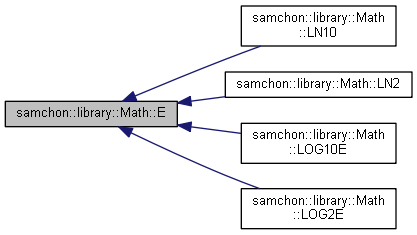static auto samchon::library::Math::PI ( ) -> double
inlinestatic

��, Number Pi

A mathematical constant for the ratio of the circumference of a circle to its diameter, expressed as pi, with a value of 3.141592653589793.

Definition at line 47 of file Math.hpp.

Here is the caller graph for this function:static auto samchon::library::Math::LN2 ( ) -> double
inlinestatic

loge2

A mathematical constant for the natural logarithm of 2, expressed as loge2, with an approximate value of 0.6931471805599453.

Definition at line 59 of file Math.hpp.

References E().

Here is the call graph for this function:static auto samchon::library::Math::LN10 ( ) -> double
inlinestatic

loge10

A mathematical constant for the natural logarithm of 10, expressed as loge10, with an approximate value of 2.302585092994046.

Definition at line 71 of file Math.hpp.

References E().

Here is the call graph for this function: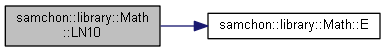static auto samchon::library::Math::LOG2E ( ) -> double
inlinestatic

log2e

A mathematical constant for the base-2 logarithm of the constant e, expressed as log2e, with an approximate value of 1.442695040888963387.

Definition at line 83 of file Math.hpp.

References E().

Here is the call graph for this function:static auto samchon::library::Math::LOG10E ( ) -> double
inlinestatic

log10e

A mathematical constant for the base-10 logarithm of the constant e (Math.E), expressed as log10e, with an approximate value of 0.4342944819032518. The Math.log() method computes the natural logarithm of a number. Multiply the result of Math.log() by Math.LOG10E to obtain the base-10 logarithm.

Definition at line 97 of file Math.hpp.

References E().

Here is the call graph for this function: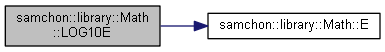static auto samchon::library::Math::SQRT1_2 ( ) -> double
inlinestatic

Squart root of 0.5.

A mathematical constant for the square root of one-half, with an approximate value of 0.7071067811865476.

Definition at line 109 of file Math.hpp.

 static auto samchon::library::Math::SQRT2 ( ) -> double
inlinestatic

Squart root of 2.

A mathematical constant for the square root of 2, with an approximate value of 1.4142135623730951.

Definition at line 121 of file Math.hpp.

 static auto samchon::library::Math::random ( ) -> double
inlinestatic

Get a random value.

Returns a random value following uniform distribution

Returns
The random value

Definition at line 135 of file Math.hpp.

Here is the caller graph for this function: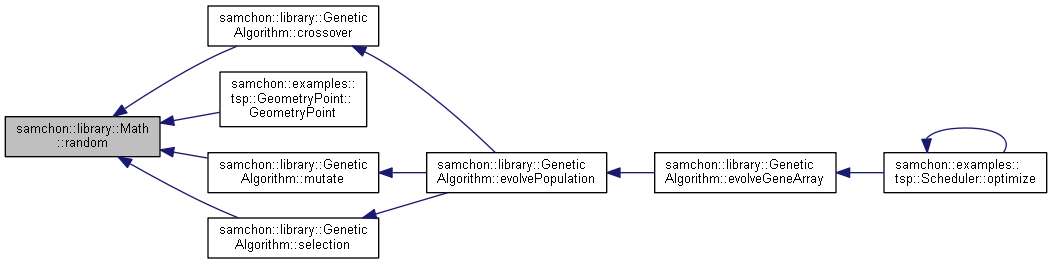static auto samchon::library::Math::degree_to_radian ( double val ) -> double
inlinestatic

Definition at line 149 of file Math.hpp.

References PI().

Referenced by samchon::examples::tsp::GeometryPoint::calcDistance().

Here is the call graph for this function:Here is the caller graph for this function:static auto samchon::library::Math::radian_to_degree ( double val ) -> double
inlinestatic

Definition at line 157 of file Math.hpp.

References PI().

Referenced by samchon::examples::tsp::GeometryPoint::calcDistance().

Here is the call graph for this function: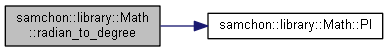Here is the caller graph for this function:template<typename _Cont , typename T = _Cont::value_type>
 static auto samchon::library::Math::minimum ( const _Cont & container ) -> IndexPair
inlinestatic

Calculate minimum value with its index.

Template Parameters
 _Cont Type of the container _Ty

Value type of the container.

Do not modify the default template argument.

Parameters
 container Container to find its minimum value
Returns
Pair of minimum value and its index

Definition at line 177 of file Math.hpp.

Here is the caller graph for this function:template<typename _Cont , typename T = _Cont::value_type>
 static auto samchon::library::Math::maximum ( const _Cont & container ) -> IndexPair
inlinestatic

Calculate maximum value with its index.

Template Parameters
 _Cont Type of the container _Ty

Value type of the container.

Do not modify the default template argument.

Parameters
 container Container to find its minimum value
Returns
Pair of maximum value and its index

Definition at line 208 of file Math.hpp.

Referenced by samchon::WeakString::rfinds(), and samchon::WeakString::rtrim().

Here is the caller graph for this function:template<typename _Cont , typename T = _Cont::value_type>
 static auto samchon::library::Math::mean ( const _Cont & container ) -> double
inlinestatic

Calculate average.

Template Parameters
 _Cont Type of the container _Ty

Value type of the container.

Do not modify the default template argument.

Parameters
 container Container to find its mean
Returns
Average(mean) of the contaier

Definition at line 239 of file Math.hpp.

Referenced by variance_p(), and variance_s().

Here is the caller graph for this function: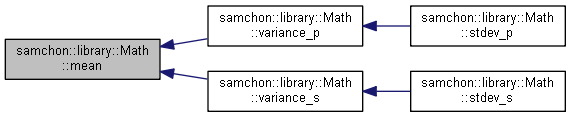template<typename _Cont , typename T = _Cont::value_type>
 static auto samchon::library::Math::median ( const _Cont & container ) -> double
inlinestatic

Get median value.

Template Parameters
 _Cont Type of the container _Ty

Value type of the container.

Do not modify the default template argument.

Parameters
 container Container to find its median
Returns
Median value of the container

Definition at line 260 of file Math.hpp.

template<typename _Cont , typename T = _Cont::value_type>
 static auto samchon::library::Math::mode ( const _Cont & container ) -> T
inlinestatic

Find mode value.

Template Parameters
 _Cont Type of the container _Ty

Value type of the container.

Do not modify the default template argument.

Parameters
 container Container to find its mode
Returns
Most appeared value in the container

Definition at line 292 of file Math.hpp.

template<typename _Cont , typename T = _Cont::value_type>
 static auto samchon::library::Math::stdev_p ( const _Cont & container ) -> double
inlinestatic

Square root of a variance_p.Template Parameters
 _Cont Type of the container _Ty

Value type of the container.

Do not modify the default template argument.

Parameters
 container Container to find its standard deviation
Returns
Standard deviation of the container

Definition at line 316 of file Math.hpp.

References variance_p().

Here is the call graph for this function: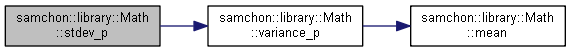template<typename _Cont , typename T = _Cont::value_type>
 static auto samchon::library::Math::stdev_s ( const _Cont & container ) -> double
inlinestatic

Square root of a variance_s.

Template Parameters
 _Cont Type of the container _Ty

Value type of the container.

Do not modify the default template argument.

Parameters
 container Container to find its variance
Returns
Variance of the container

Definition at line 327 of file Math.hpp.

References variance_s().

Here is the call graph for this function: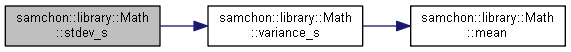template<typename _Cont , typename T = _Cont::value_type>
 static auto samchon::library::Math::variance_p ( const _Cont & container ) -> double
inlinestatic

Calculate standard deviation for all population.

Template Parameters
 _Cont Type of the container _Ty

Value type of the container.

Do not modify the default template argument.

Parameters
 container Container to find its standard deviation
Returns
Standard deviation of the container

Definition at line 344 of file Math.hpp.

References mean().

Referenced by stdev_p().

Here is the call graph for this function:Here is the caller graph for this function: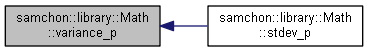template<typename _Cont , typename T = _Cont::value_type>
 static auto samchon::library::Math::variance_s ( const _Cont & container ) -> double
inlinestatic

Calculate variance for sample.

Template Parameters
 _Cont Type of the container _Ty

Value type of the container.

Do not modify the default template argument.

Parameters
 container Container to find its variance
Returns
Variance of the container

Definition at line 367 of file Math.hpp.

References mean().

Referenced by stdev_s().

Here is the call graph for this function: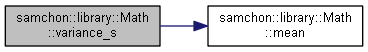Here is the caller graph for this function: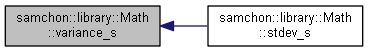The documentation for this class was generated from the following file:
• D:/OneDrive/Project/Samchon/framework/cpp/samchon/library/Math.hpp